Let's Get Started

Let's use what we know about slope to make predictions about how a change will affect the slope of the function describing that situation.

TEKS Standards and Student Expectations

A(3) Linear functions, equations, and inequalities. The student applies the mathematical process standards when using graphs of linear functions, key features, and related transformations to represent in multiple ways and solve, with and without technology, equations, inequalities, and systems of equations. The student is expected to:

A(3)(E) determine the effects on the graph of the parent function f(x) = x when f(x) is replaced by af(x), f(x) + d, f(x - c), f(bx) for specific values of a, b, c, and d

Resource Objective(s)

Given verbal, symbolic, numerical, or graphical representations of problem situations, the student will interpret and predict the effects of changing the slope in the context of the situations.

Essential Questions

How does changing the slope affect the graph of a function?

How does changing the slope affect the table of values for a function?

How does changing the slope affect the equation of a function?

Vocabulary

Introduction

Linear functions can appear in a variety of representations.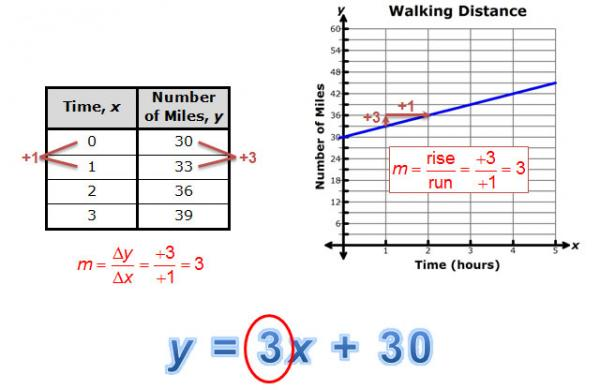Regardless of the representation, you can determine the value of the slope when it describes a problem situation.

In the examples shown, the slope of 3 can be interpreted as the number of miles walked in each hour. In other words, the family is walking at a rate of 3 miles per hour.Interpreting and Predicting Changes in Slopes from a Graph

The slope (m) and the y-intercept (b) have an affect on the graph of y = mx + b. The slope (m) affects the steepness of the graph, and the y-coordinate of the y-intercept (b) affects where the graph crosses the y-axis.

Example

Alyssa received $30 from her favorite aunt for her birthday. She wants to save her money to purchase an e-reader. She decided to save the$30 and add \$5 from her own money each week. The amount of money that she has saved as a function of time is shown in the graph below.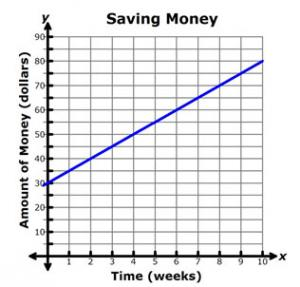Check your knowledge by interpreting these three graphs and answering the associated questions.

Interpreting and Predicting Changes in Slopes from a Table

You can also use a table of values to interpret and make predictions about the changes in the slopes of linear functions.

Example

An electrician charges a fixed service fee plus an hourly rate for labor, in addition to the cost of the parts for the electrical repair.

Miguel’s Electric Company uses a table like the one shown to determine how much to charge for labor.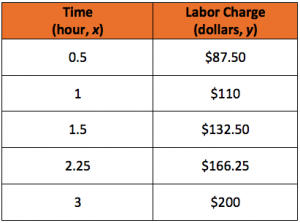Hallie is tracking the amount of money in her savings account and how it changes weekly. Observe the following table and answer the three questions that present different scenarios.

Interpreting and Predicting Changes in Slopes from an Equation

Another representation commonly used for linear relationships is an equation, or symbolic representation.

The Rimrock Trail in Balcones Canyonlands National Wildlife Refuge in central Texas begins at an elevation of 1,100 feet at the base along the creek bed and ascends to an elevation of 1,300 feet at the top of the canyon.The Hernandez family used the following equation to describe their elevation in feet above sea level, y, as a function of time since the hike began, x.Summary

We've now investigated how to interpret changes in slopes and rates of change for linear functions using graphs, tables, and equations.

Consider the graphs, equations, and tables shown below. Each pair of representations compares draining a pool with 12 feet of water at a rate of 1.5 feet per hour to draining a pool with 12 feet of water at the same rate of 1.25 feet per hour.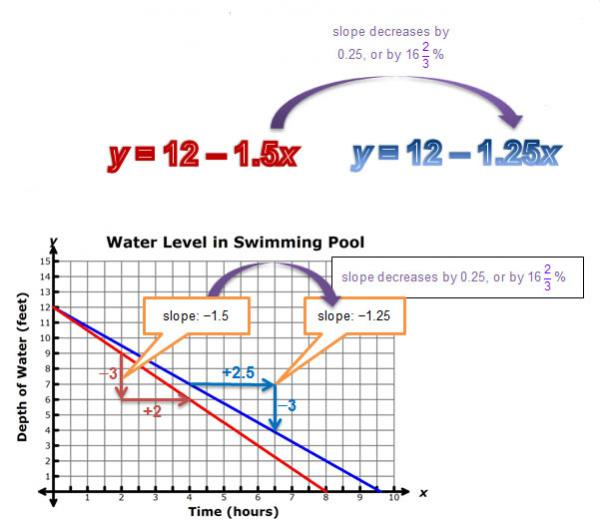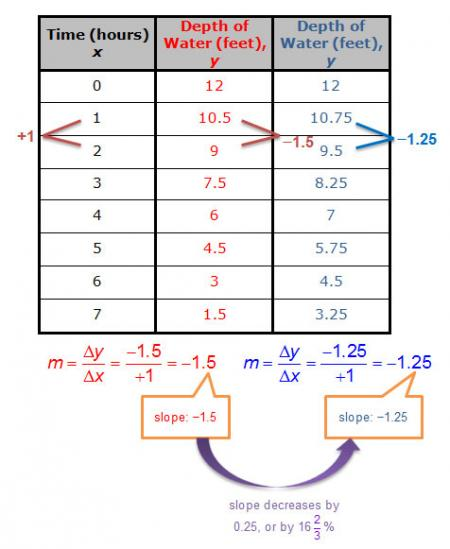In each representation, the original slope of −1.5 feet per hour changes to −1.25 feet per hour, which is a decrease of 0.25 feet per hour, or 16 2/3% from the original value.